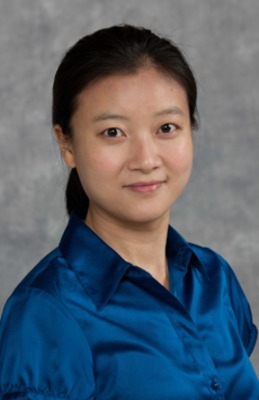## Academic Year 2015-2016

#### Description

This presentation will start by a general introduction of Bayesian statistics, which has become popular in the era of big data. Then we consider a two-class classification problem, where the goal is to predict the class membership of M units based on the values of high-dimensional categorical predictor variables as well as both the values of predictor variables and the class membership of other N independent units. We focus on applying generalized linear regression models with Boolean expressions of categorical predictors. We consider a Bayesian and decision-theoretic framework, and develop a general form of Bayes multiple binary classification functions with respect to a class of cost-weighted loss functions. In particular, the loss function pairs such as the proportions of false positives and false negatives, and (1-sensitivity) and (1-specificity), are considered. The results will be illustrated via simulations and on a Lupus diagnosis dataset.

#### Presenter Bio

Wensong Wu received a BS in computational mathematics from Nanjing University, China in 2004, and MS and PhD in statistics from University of South Carolina in 2007 and 2011, respectively. She joined FIU as an Assistant Professor in the Division of Statistics, Department of Mathematics and Statistics at Florida International University in 2011. Her research interest includes Bayesian decision theory in classification, model selection and model averaging, and statistical learning theory and applications

#### Presenter Profile Page(s)

http://mathstat.fiu.edu/faculty/wensong-wu/

#### Date of Event

November 10, 2015 12:00-1:00 PM

#### Location

Mailman-Hollywood Auditorium

#### Share

COinSNov 10th, 12:00 PM Nov 10th, 1:00 PM

Bayes Multiple Binary Classifier - How to Make Decisions Like a Bayesian

Mailman-Hollywood Auditorium

This presentation will start by a general introduction of Bayesian statistics, which has become popular in the era of big data. Then we consider a two-class classification problem, where the goal is to predict the class membership of M units based on the values of high-dimensional categorical predictor variables as well as both the values of predictor variables and the class membership of other N independent units. We focus on applying generalized linear regression models with Boolean expressions of categorical predictors. We consider a Bayesian and decision-theoretic framework, and develop a general form of Bayes multiple binary classification functions with respect to a class of cost-weighted loss functions. In particular, the loss function pairs such as the proportions of false positives and false negatives, and (1-sensitivity) and (1-specificity), are considered. The results will be illustrated via simulations and on a Lupus diagnosis dataset.